Mathematics

# Is Calculus Hard ?

273 views

## Table of Contents

 1 Introduction 2 Is calculus hard? 3 How hard is calculus? 4 Calculus is hard! But interesting! 5 Summary

26 November 2020

Read time: 5 minutes

## Introduction

When Ramanujam returned from the office, he was used to seeing his daughter Ratna waiting to tell him all that she had learned in school.

Today she was in her room and that meant that something was not quite right. He decided to have his evening coffee with Ratna.Distracted playing with the hair, the pencil end chewed …again and that frown!

Ramanujam: “So, what’s bothering you princess?” asked Ramanujam

Ratna: “Papa! Is Calculus hard? And how hard is it? This math is driving me crazy! Why is calculus so hard?”

Ramanujam peeped into the paper Ratna had been scribbling on, “oh, it’s driving you cal-cu-lus, you mean?”

Ratna: “C’mon papa! I am so confused! How hard is calculus!”

Ramanujam: “Is Calculus hard?” Ramanujam repeated slowly and thoughtfully.

Ramanujam pulled up a chair and sat down “Ratna tell me what is geometry?”

Ratna: “Geometry? Angles, triangles, squares, circles…….”

Ramanujam: You mean shapes, right? Is there anything else that you study in geometry?

Ratna: “Ah! Spaces!”

Ramanujam: “That’s my girl! What about Algebra?”

Ratna smiling broadly: “Lazy man’s math. You pretend you know what you don’t know by calling it names like “x” “y” “z”!”

Ramanujam: “Wow! You remembered that tip! And what is Probability?

Ratna: (scratching her head) “when many things can happen, when there is a lot of choices”Also read:

## Is Calculus Hard?

Ramanujam: “Now, Ratna, who is your favorite cricketer?

Ratna: “Kohli of course! But Papa, we were discussing how hard is Calculus!”

Ramanujam: “Be patient Ratna, I am trying to answer your question- is Calculus hard? Ok…that er….Virat Kohli, imagine that he bowls to a batsman. Can you calculate the time taken by the ball to hit the bat of the batsman?”

Ratna: “Yes. We need to note the time when the ball left the bowlers and hand and the time when it hit the bat”

Ramanujam: “ You know the distance traveled by the ball too as you know the length of the pitch. Right?”

Ratna nods her head

Ramanujam: “ what was the path of the ball like? Was it straight?

Ratna: “no, it went as a curve”

Ramanujam: “Good! So, what was the speed of the ball when it was halfway the pitch? or what was the speed of the ball when it had traveled $$\frac{3}{4}^{th}$$ of the curve?”

Ratna: That’s difficult, the distance traveled by the ball and its speed would have changed so many times and so many other factors too like a breeze, how tired Kohli is…etc

Ramanujam: “Ratna, now let me answer your question is Calculus Hard? First, understand what Calculus is: Calculus is about calculating CHANGE. It is the Mathematics of change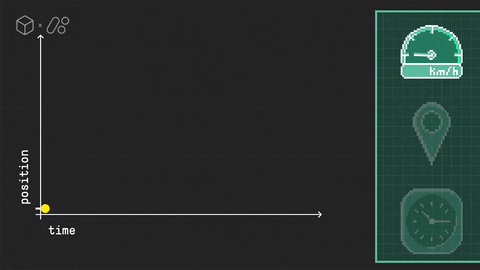Source: giphy.com

Ratna: WOW! But there are so many things changing around us. Speed of vehicles, weather, prices of commodities, population, and now these COVID infected numbers….Do you mean to say we can calculate all that using Calculus? My God! How hard is Calculus!?

Ramanujam:Calculus is easy like any topic in Mathematics. You just need to know the previous topics you have covered in maths and be ready to apply those concepts to learning Calculus.

As you said, there are so many things constantly changing around us. Using Calculus we can study the pattern of change and predict the time taken to reach a destination, population in 2030, number of Expected COVID cases by December 2020., etc.

## How hard is Calculus?

Ratna: that means there is a pattern to all the changes happening around us? So many things are changing, God, this is scary! How hard is Calculus, Papa?

Ramanujam: Hear me out Ratna, and then you can decide for yourself- is Calculus hard? Or Calculus is easy!

In Calculus, we study the pattern of change and this pattern is called FUNCTION in Calculus. To understand these functions we use three tools- Limits, differentiation, and integration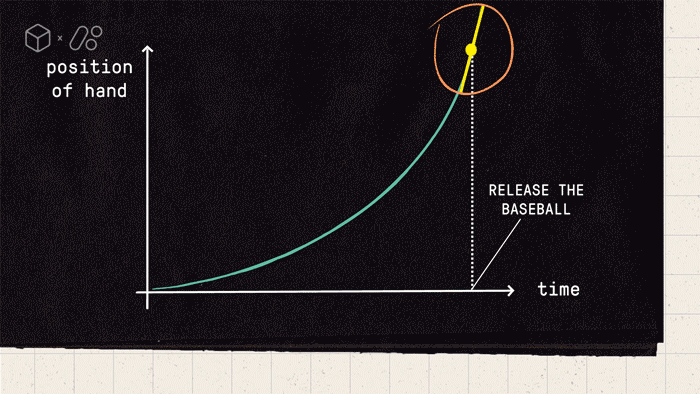Source: giphy.com

Ratna: Hmmm….

Ramanujam: Now you have a conception in your mind and that is why you asked me, why is calculus so hard?

Coming back to your favorite, Virat Kohli, Do you know the speed of the ball midway as it traveled to the batman?

Ratna: The average speed of the ball?

Ramanujam: Are you sure that the speed was constant? Didn’t it change from the time it left Kohli’s hands and curved all the way to hit the ground?

Ratna: I am sure it would have changed all along! It’s not possible to tell the speed at different times, is it?

Ramanujam: With calculus it is possible. Calculus helps you to calculate the speed of the ball at any time on the curve. Calculus also tells you the angle one needs to throw the ball so that the ball covers maximum distance.

Now let me ask you another question - You know the formula for the area of a triangle, square, circle, etc. How are these shapes similar?

Ratna: Mmmmmm…..they have a definite shape.

Ramanujam: Good! But what about the area of a jug, dome, a hillside surface, etc.? They don’t have a definite shape, isn’t it?

Ratna: oh yes! I never thought about that. Are you telling me Calculus has a solution to this too?

Ramanujam: Of course! Since the shape is irregular, its area will change. But there are certain concepts of calculus. E.g Infinity. Let me give you an example: Let’s take a square – I m length.

Let us divide the square into parts as shown in the figure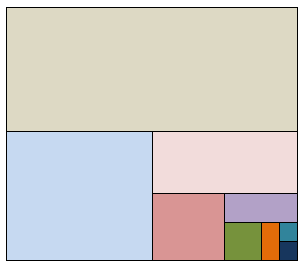The formula tells us that the area is $$\text{1 m} \times \text{1m = 1 Sqm}$$

But, if we were to divide this square, as shown above, we could write it as:

$1= \frac{1}{2}+ \frac{1}{4}+ \frac{1}{8} +\frac{1}{16}+ \frac{1}{32}+\frac{1}{64}+\frac{1}{128} +\frac{1}{256} ………$

This means there are infinite parts to that “1” the parts will get smaller and smaller till it ALMOST reaches 0 but NEVER 0.

In other words, we can split a number into INFINITE parts before it reaches a DEFINITE number

Ratna: Hmmm this also means that we can calculate values at different stages. Like the speed of Kohli’s ball midway through the pitch!

Ramanujam: Exactly! This is known as differentiation.- one of the principles of Calculus. This is useful to analyze the rate of change of a certain value in a situation.

Remember, we just discussed objects that don’t have a definite shape? Let us see how the area of such shapes is calculated using Calculus. So, Ratna, how hard is Calculus?

## Calculus is hard! But interesting!

Ratna: Calculus is hard! But interesting!

Ramanujam: Ancient people were comfortable calculating the area of objects with a definite shape. But when it came to a circle they were a little confused. So they put the circle in a square and another square in the circle and decided that the area of the circle will be between that of the area between two squares.

On second thoughts they felt that using a polygon in place of a square would be a better idea. In the case of a polygon, they could keep increasing the area until they got an almost accurate area of the circle.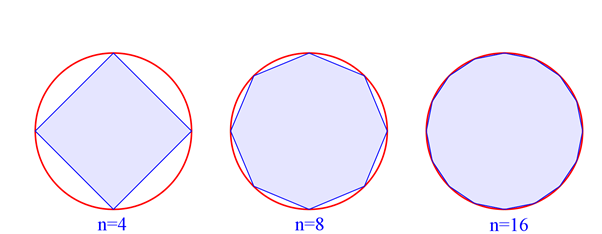We know that area of the polygon will always be less than the area of the circle because if it were not less than the circle it would no longer be able to be inscribed in the circle as the area of an inscribed figure is always less than the area of the figure circumscribing it.

Therefore we can say that the area of the polygon is limited by the area of the circle.

This is called the method of exhaustion. It is a method for finding the area of a shape by inscribing regular polygons inside of it with an increasing number of sides. So hard calculus problems can be simplified in a similar way.

As the number of sides to the polygons increases the area of the polygons converge to the area of the shape in which the polygons are inscribed.

But there was a problem here. For every irregular shape, we needed to figure out which shape to sandwich it with so that we get an idea about the area. So, the solution was RECTANGLES!

So, coming back to the ball thrown by Kohli, from the beginning part of a curve on the Y axis, to the last point on the curve we could draw multiple/ infinite rectangles.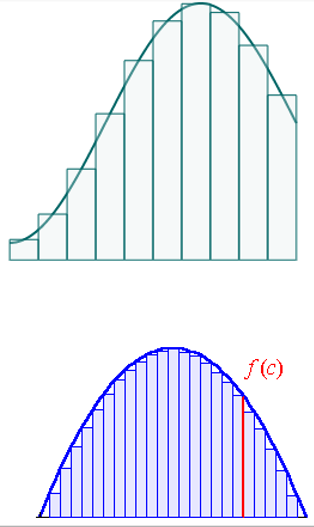These rectangles could be either above the curve or enclosed in the curve. Since we can make an infinite number of rectangles we can say that the difference between the rectangles outside the square and the difference between rectangles inside the square would become lesser and lesser till it reaches the exact area of the square.

This process is called integration.

Ratna: Wow! This is so interesting! So, this is how we measure the area covered by dome-like in stadiums!

## Is Calculus Hard? - PDF

If you ever want to read it again as many times as you want, here is a downloadable PDF to explore more.

 📥 Is Calculus Hard? - PDF Download

Here are some more of our articles:

## Summary

Ramanujam: Yes! So, How hard is Calculus?

Ratna: Well, Calculus is hard but really interesting! Especially when it seems to be used all around me!

Ramanujam: Yes Ratna. You need to be thorough in Algebra, geometry, and trigonometry then you will be able to understand the concepts in Calculus and apply it too and that will make it seem like Calculus is easy.

Remember maths is like a building block. It builds on what you have already been taught. To enjoy Mathematics one needs to revisit the basics and make the foundation knowledge of Maths really strong, then the tougher concepts will be easy to comprehend.

Ratna: Thank you papa for explaining all this to me. Instead of asking around why is calculus so hard, and is calculus hard, now I am ready to take on the challenge of learning and applying Calculus, the Mathematics of change.

A lot of topics in mathematics can seem hard, even harder than expected if you don't put in regular and consistent work. Understanding, learning, and practicing the concepts are all essential to get better at mathematics. Getter at mathematics with Cuemath sign up for a free trial.

Written by Jayashree Nair

## About Cuemath

Cuemath, a student-friendly mathematics and coding platform for academics and skill-development, and their Mental Math App, on both iOS and Android, is a one-stop solution for kids to develop multiple skills. Understand the Cuemath Fee structure and sign up for a free trial.

## Why people fail Calculus?

There are a few reasons why you may not be doing so well in calculus:

• You and the professor have different expectations
• You're not doing enough homework problems or you're not doing the right homework problems
• Weak algebra and trigonometry skills

## What is calculus used for?

Calculus is used for numerous things in science, here are a few examples:

• Finding the areas of shapes
• Engineering and architecture to determine the shapes of structures and curves
• In Economics, it is used to determine the right time to buy and sell products
• Biologists use calculus to determine the growth rate of bacteria

Watch this short and simple video to better understand the uses of calculus:

## External References

GIVE YOUR CHILD THE CUEMATH EDGE
Access Personalised Math learning through interactive worksheets, gamified concepts and grade-wise courses
Learn More About Cuemath